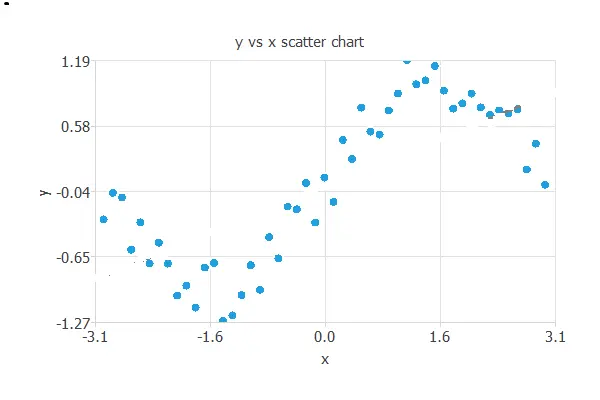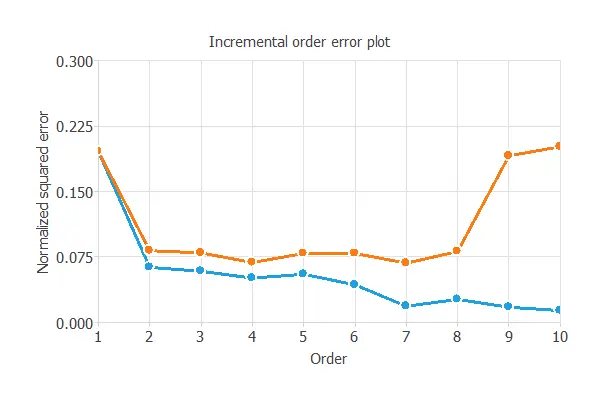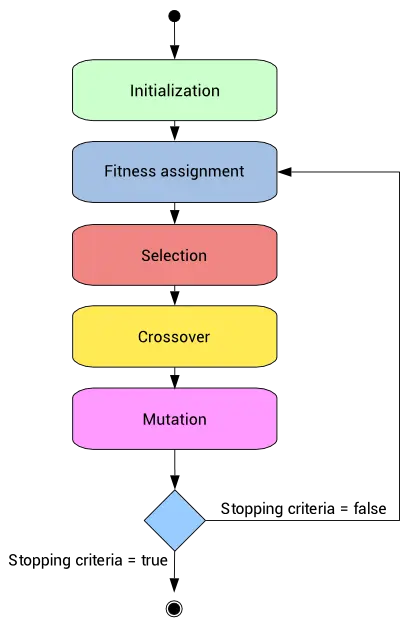# 5. Model selectionThe objective of model selection is to find the network architecture with the best generalization properties, that is, that which minimizes the error on the selected instances of the data set (the selection error).

There are two families of model selection algorithms:

## 5.1. Neurons selection

Two frequent problems in designing a neural network are called underfitting and overfitting. The best generalization is achieved by using a model with the most appropriate complexity to produce a good data fit.

To illustrate underfitting and overfitting, consider the following data set. It consists of data taken from a sine function to which white noise has been added.The best generalization is achieved by using a model whose complexity is the most appropriate to produce an adequate fit of the data. In this case, we use a neural network with one input (x), three hidden neurons, and one output (y).In this way, underfitting is defined as the effect of a selection error increasing due to a too-simple model. Here we have used one hidden neuron.On the contrary, overfitting is defined as the effect of a selection error increasing due to a very complex model. In this case, we have used 10 hidden neurons.The error of a neural network on the training instances of the data set is called the training error. Similarly, the error on the selected instances is called the selection error.

The training error measures the ability of the neural network to fit the data that it sees. But the selection error measures the ability of the neural network to generalize to new data.

The following figure shows the training (blue) and selection (orange) errors as a function of the neural network complexity,
represented by the number of hidden neurons.As we can see, the more hidden neurons, the smaller the training error. However, for small and big complexities, the selection error is significant. In the first case, we have underfitting, and in the second one, overfitting. In our case, the neural network with the best generalization properties has four hidden neurons. Indeed, the selection error takes a minimum value at that point.

Neurons selection algorithms are used to find the neural network’s complexity, yielding the best generalization properties.
The most used algorithm is the growing neurons.

### Growing neurons

Growing neurons is the most straightforward neurons selection algorithm. This method starts with small neurons and increases the complexity until any stopping criterion is met.The algorithm returns the neural network with the optimal number of neurons obtained.

## 5.2. Inputs selection

Which features should you use to create a predictive model? This is a difficult question that may require in-depth knowledge of the problem domain.

Input selection algorithms automatically extract those features in the data set that provide the best generalization capabilities.
They search for the subset of inputs that minimizes the selection error.

The most common input selection algorithms are:

### Growing inputs

The growing inputs method calculates the correlation of every input with every output in the data set.

It starts with a neural network that only contains the most correlated input and calculates the selection error for that model.

It keeps adding the most correlated variables until the selection error increases.The algorithm returns the neural network with the optimal subset of inputs found.

### Pruning inputs

The pruning inputs method starts with all the inputs in the data set.

It keeps removing those inputs with the smallest correlation with the outputs until the selection error increases.### Genetic algorithm

A different class of inputs selection method is the genetic algorithm.

This is a stochastic method based on natural genetics and biological evolution mechanics. Genetic algorithms usually include fitness assignment, selection, crossover, and mutation operators.